# 数学建模的介绍与作用

• 培养科学研究的能力
• 培养通过研究学习新认识的能力
• 培养团队合作能力
• 培养创新意识和创造能力
• 训练快速获取信息和资料的能力
• 锻炼快速了解和掌握新知识的技能
• 培养团队合作意识和团队合作精神
• 增强写作技能和排版技术
• 更重要的是训练人的逻辑思维和开放性思考方式

# 1 三大模型与十大常用算法【简介】

## 1-1 三大模型

 预测模型：神经网络预测、灰色预测、拟合插值预测（线性回归）、时间序列预测、马尔科夫链预测、微分方程预测、Logistic 模型等等。

 优化模型：规划模型（目标规划、线性规划、非线性规划、整数规划、动态 规划）、图论模型、排队论模型、神经网络模型、现代优化算法（遗传算法、模拟退火算法、蚁群算法、禁忌搜索算法）等等。

 评价模型：模糊综合评价法、层次分析法、聚类分析法、主成分分析评价法、

## 1-2 十大常用算法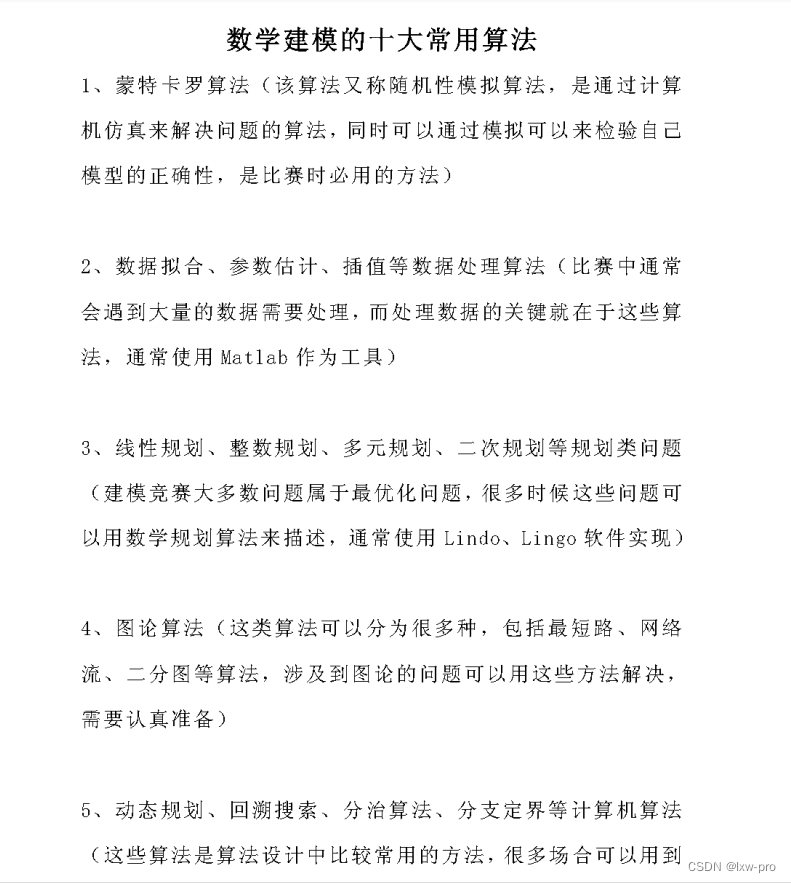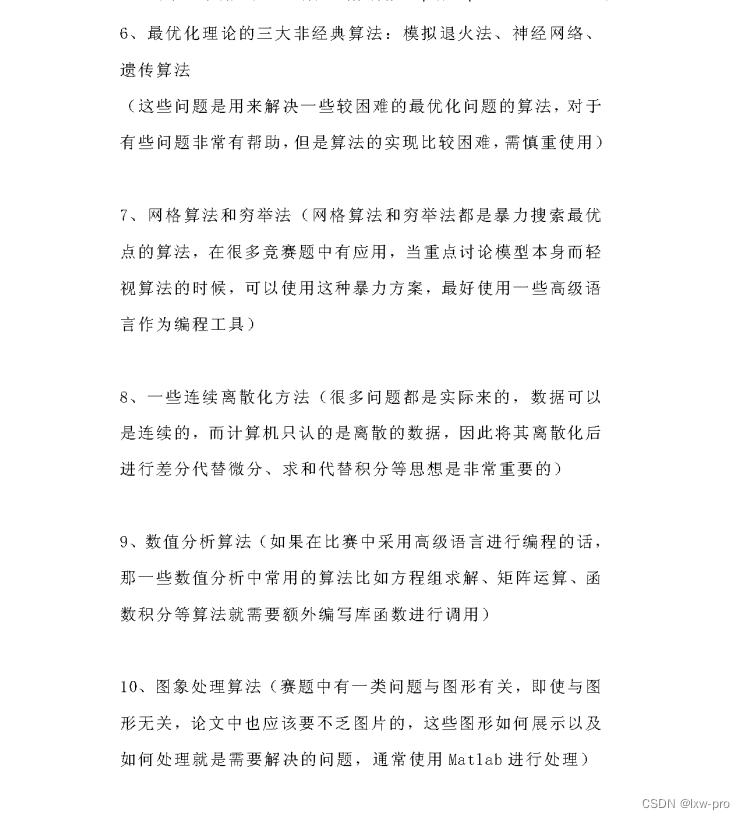# 2 python数据分析之Pandas

## 2-1什么是pandas

· 高性能
· 容易使用的数据结构
· 容易使用的使用分析工具

· numpy:用于科学计算
· scikit-learn:用于机器学习

## 2-2 pandas读取文件

'''

Pandas提供了多种读取数据的方法：

1-导入库import pandas as pd
2-找到文件所在位置（绝对路径=全称）（相对路径=和程序在同一个文件夹中的路径的简称）
3-变量名=pd.读写操作方法（文件路径，具体的筛选条件，...）

./  当前路径
../ 上一级

DataFrame中。

'''

import pandas as pd

print(df, type(df))
# Pandas默认使用utf-8读取文件

print()
import pandas as pd

lxw = open(r"t.csv", encoding='utf-8')

print()
import os

# 打印当前目录
print(os.getcwd())



Pandas需要先读取表格类型的数据，然后进行分析

【详细版学习】

# 1:
import pandas as pd

print(df)

# 2:
import pandas as pd

# to_string() 用于返回 DataFrame 类型的数据，如果不使用该函数，则输出结果为数据的前面 5 行和末尾 5 行，中间部分以 ... 代替(如上）
print(df.to_string())

# 3:
import pandas as pd

# 三个字段 name, site, age
nme = ["Google", "Runoob", "Taobao", "Wiki"]
st = ["www.google.com", "www.runoob.com", "www.taobao.com", "www.wikipedia.org"]
ag = [90, 40, 80, 98]

# 字典
dict = {'name': nme, 'site': st, 'age': ag}

df = pd.DataFrame(dict)

# 保存 dataframe
print(df.to_csv('site.csv'))

# 4:
import pandas as pd

# head( n ) 方法用于读取前面的 n 行，如果不填参数 n ，默认返回 5 行
df.tail(10).to_csv('site4.csv')
print("over!")

# 5:
import pandas as pd

# 读取前面 10 行

# 6:
import pandas as pd

# tail( n ) 方法用于读取尾部的 n 行，如果不填参数 n ，默认返回 5 行，空行各个字段的值返回 NaN
print(df.tail())

# 7:
import pandas as pd

#  读取末尾 10 行
print(df.tail(10))

# 8:
import pandas as pd

# info() 方法返回表格的一些基本信息
print(df.info())

# non-null 为非空数据，我们可以看到上面的信息中，总共 458 行，College 字段的空值最多



所有csv、excel文件可在资源当中自行提用，没有的可自行补充！
提示：须提前安装好pandas库。【终端安装命令：pip install pandas】

# -*- coding = utf-8 -*-
# @Time : 2022/6/27 11:45
# @Author : lxw_pro
# @File : pandas的介绍-1.py
# @Software : PyCharm
import pandas as pd

# 查看前几行数据

# 查看索引列
print(lxw.index)

# 查看列名列表
print(lxw.columns)

# 查看数据的形状（返回行、列数）
print(lxw.shape)

# 查看每列的数据类型
print(lxw.dtypes)

print()
# 读取txt文件，自己指定分隔符、列名
fpath = 'D:\PyCharm\数学建模大赛\数据分析-上-2\Python成绩.csv'

fpath,
sep=',',
names=['name', 'Python-score']
)

# print(lxw)
lxw.to_csv('Python成绩2.csv')

# 读取excel文件：
import pandas as pd

print(lxw)



[^1]注：如果最后这里【读取excel文件]运行出错，那就在终端安装 pip install xlrd

## 2-3 pandas数据结构

'''
1-Series：  一维数据，一行或一列
【Series是一种类似于一维数组的对象，它由一组数据（不同数据类型）以		    及一组与之相关的数据标签（即索引）组成】

2-DataFrame:二维数据，整个表格，多行多列

'''

import pandas as pd

# 1-1仅用数据列表即可产生最简单的Series
lxw = pd.Series([1, 'a', 5.2, 6])

print(lxw)      # 运行结果解说：左边为索引，右边为数据

# 获取索引
print(lxw.index)

# 获取数据
print(lxw.values)

print()
# 1-2 创建一个具有索引标签的Series
lxw2 = pd.Series([5, '程序人生6', 666, 5.2], index=['sz', 'gzh', 'jy', 'xy'])

print(lxw2)
print(lxw2.index)

# 写入文件当中
lxw2.to_csv('gzh.csv')

print()
# 1-3 使用过Python字典创建Series
lxw_ej = {'python': 390, 'java': 90, 'mysql': 90}

lxw3 = pd.Series(lxw_ej)
print(lxw3)

# 1-4 根据标签索引查询数据
print(lxw3['java'])

print(lxw2['gzh'])
print(lxw2[['gzh', 'jy']])
print(type(lxw2[['gzh', 'jy']]))

print(lxw)
print(type(lxw))

print()
# 2 根据多个字典序列创建dataframe
lxw_cj = {
'ps': [86, 92, 88, 82, 80],
'windows操作系统': [84, 82, 88, 80, 92],
'网页设计与制作': [92, 88, 97, 98, 83]
}

df = pd.DataFrame(lxw_cj)

# print(df)
# df.to_excel('lxw_cj.xlsx')      # 须提前安装好openxlsx,即pip install openpyxl[可在终端安装]
print("over!")

print(df.dtypes)

print(df.columns)

print(df.index)

print()
# 3-从DataFrame中查询Series
'''
·如果只查询一行、一列的话，那么返回的就是pd.Series
·如果查询多行、多列时，返回的就是pd.DataFrame

'''

# 一列：
print(df['ps'])
print(type(df['ps']))

# 多列：
print(df[['ps', 'windows操作系统']])
print(type(df[['ps', 'windows操作系统']]))

print()
# 一行：
print(df.loc)
print(type(df.loc))

# 多行：
print(df.loc[1:3])
print(type(df.loc[1:3]))



DataFrame 加强

### 2-3-1 pandas数据结构之DataFrame

# DataFrame数据类型
'''
DataFrame是Pandas的重要数据结构之一，也是在使用数据分析过程中最常用的结构之一，

'''

# 认识Dataframe结构：
'''
Dataframe是一个表格型的数据结构，既有行标签，又有列标签，她也被称异构数据表，所谓

Dataframe的每一列数据都可以看成一个Series结构，只不过，Dataframe为每列数据值增加了

'''

# 特点：
'''
Dataframe   每一列的标签值允许使用不同的数据类型；
Dataframe   是表格型的数据结构，具有行和列；
Dataframe   中的每个数据都可以被修改
Dataframe   结构的行数、列数允许增加或者删除
Dataframe   有两个方向的标签轴，分别是行标签和列标签
Dataframe   可以对行和列执行算术运算

'''

# DataFrame 构造方法如下：

# pandas.DataFrame( data, index, columns, dtype, copy)
'''
data：输入的数据，可以是ndarray, series, list, dict, 标量以及一个Dataframe；

index：行标签，如果没有传递index值，则默认行标签是RangeIndex(0, 1, 2, ..., n)代表data的元素个数；

columns：列标签，如果没有传递columns值，则默认列标签是RangIndex（0, 1, 2, ..., n)；

dtype:要强制的数据类型，只允许使用一种数据类型，如果没有，自行推断；

copy:从输入复制数据。对于dict数据, copy=True, 重新复制一份。对于Dataframe或者ndarray输入，类似于copy=False,它用的是试图。

'''

# 1: 使用普通列表创建
import pandas as pd

lxw = [5, 2, 1, 3, 1, 4]
df = pd.DataFrame(lxw)
df2 = pd.Series(lxw)
print(df)
print(df2)

print()
# 2:使用嵌套列表创建
import pandas as pd

lxw = [['lxw', 21], ['cw', 23], ['tzs', 22]]
df3 = pd.DataFrame(lxw, columns=['Name', 'Age'])
print(df3)

# 指定数值元素的数据类型为float
# 注：dtype只能设置一个，设置多个列的数据类型，需要使用其他公式

print()
# 分配列标签注意点
import pandas as pd

# 分配列标签
lxw2 = [['lxw', '男', 21, 6666], ['cw', '女', 22, 6520], ['ky', '女', 20, 5200], ['tzs', '男', 22, 6523]]
# int满足某列特征，会自动使用，不满足，则会自动识别
df = pd.DataFrame(lxw2, columns=['Name', 'xb', 'age', 'gz'], dtype=int)
print(df)

print(df['Name'].dtype)

print()
# ~字典创建：
import pandas as pd

lxw3 = [{'a': 1, 'b': 2}, {'a': 5, 'b': 8, 'c': 9}]
df = pd.DataFrame(lxw3, index=['first', 'second'])

print(df)
# 注：如果其中某些元素缺失，也就是字典的key无法找到对应的value将使用NaN代替

print()
# 使用列表嵌套字典创建一个DataFrame对象
import pandas as pd

# lxw3
df1 = pd.DataFrame(lxw3, index=['first', 'second'], columns=['a', 'b'])

df2 = pd.DataFrame(lxw3, index=['first', 'second'], columns=['a', 'b2'])

print(df1)
print("============================================")
print(df2)


import pandas as pd

data = [['lxw', 10], ['wink', 12], ['程序人生6', 13]]

df = pd.DataFrame(data, columns=['Site', 'Age'], dtype=float)

print(df)

# 1：使用 ndarrays 创建
import pandas as pd

data = {'Site': ['lxw', '程序人生6', 'wink'], 'Age': [10, 12, 13]}

df = pd.DataFrame(data)

print(df)

# 2：还可以使用字典（key/value），其中字典的 key 为列名:
import pandas as pd

data = [{'a': 1, 'b': 2}, {'a': 5, 'b': 10, 'c': 20}]

df = pd.DataFrame(data)

print(df)
# 没有对应的部分数据为 NaN

# 3：Pandas 可以使用 loc 属性返回指定行的数据，如果没有设置索引，第一行索引为 0，第二行索引为 1，以此类推：
import pandas as pd

data = {
"calories": [420, 380, 390],
"duration": [50, 40, 45]
}

# 数据载入到 DataFrame 对象
df = pd.DataFrame(data)

# 返回第一行
print(df.loc)
# 返回第二行
print(df.loc)

print(df.loc)

# 注意：返回结果其实就是一个 Pandas Series 数据。
# 也可以返回多行数据，使用 [[ ... ]] 格式，... 为各行的索引，以逗号隔开：



### 2-3-1 Pandas 数据结构之Series

# Pandas Series 类似表格中的一个列（column），类似于一维数组，可以保存任何数据类型。
'''
Series 由索引（index）和列组成，函数如下：

pandas.Series( data, index, dtype, name, copy)

data：一组数据(ndarray 类型)。

index：数据索引标签，如果不指定，默认从 0 开始。

dtype：数据类型，默认会自己判断。

name：设置名称。

copy：拷贝数据，默认为 False。

'''

import pandas as pd

lxw = [1, 2, 3]
myvar = pd.Series(lxw)
print(myvar)

print()
# 如果没有指定索引，索引值就从 0 开始，我们可以根据索引值读取数据
print(myvar)

print()
import pandas as pd

myvar2 = pd.Series(lxw, index=['x', 'y', 'z'])
print(myvar2)

print()
# 根据索引值读取数据:
print(myvar2['y'])

print()
# 也可以使用 key/value 对象，类似字典来创建 Series
import pandas as pd

lxw = {1: "Google", 2: "Runoob", 3: "Wiki"}
myvar3 = pd.Series(lxw)
print(myvar3)

print()
# 只需要字典中的一部分数据，只需要指定需要数据的索引即可
myvar3 = pd.Series(lxw, index=[1, 2])
print(myvar3)

print()
# 设置 Series 名称参数
import pandas as pd

lxw = {1: "Google", 2: "Runoob", 3: "Wiki"}
myvar4 = pd.Series(lxw, index=[1, 3], name="lxw-pro")
print(myvar4)



## 2-4查询数据

# -*- coding = utf-8 -*-
# @Time : 2022/6/27 13:16
# @Author : lxw_pro
# @File : pandas查询数据-4.py
# @Software : PyCharm

# Pandas查询数据的四种方法：
'''
1-df.loc方法，根据行、列的标签值查询
2-df.iloc方法，根据行、列的数字位置查询
3-df.where方法
4-df.query方法

'''

# Pandas使用df.loc查询数据的方法：
'''
1-使用单个label值查询数据
2-使用值列表批量查询
3-使用数值区间进行范围查询
4-使用条件表达式查询
5-调用函数查询

'''

# 注：以上方法，即适用于行，也使用于列

import pandas as pd

df.set_index('create_dt', inplace=True)

# print(df.index)
a = df.index

# 去重->转为列表->排顺序
qc = sorted(list(set(a)))
# print(qc)

# 替换掉利润率当中的后缀%
df.loc[:, 'lrl'] = df['lrl'].str.replace("%", "").astype('int32')   # astype()    对数据类型进行转换



**

astype()相关知识阐述:

**

'''
Python中与数据类型相关函数及属性有如下三个：type/dtype/astype

type()    返回参数的数据类型

dtype    返回数组中元素的数据类型

astype()    对数据类型进行转换

'''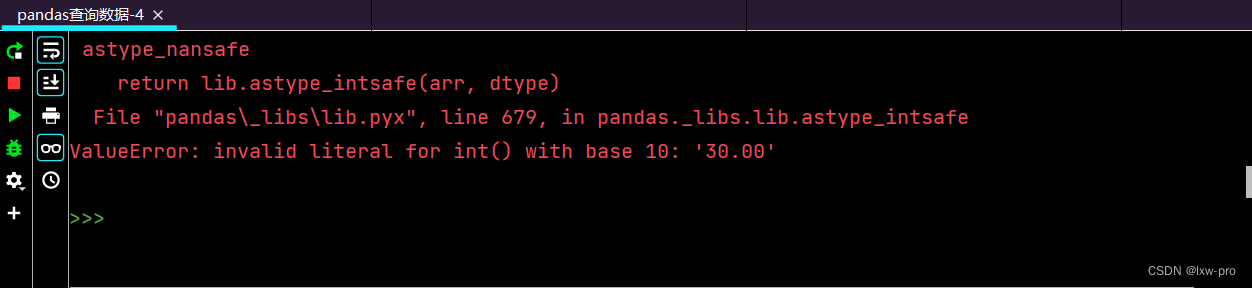# 替换掉利润率当中的后缀%
df['lrl'] = df['lrl'].map(lambda x: x.rstrip('%'))
print(df)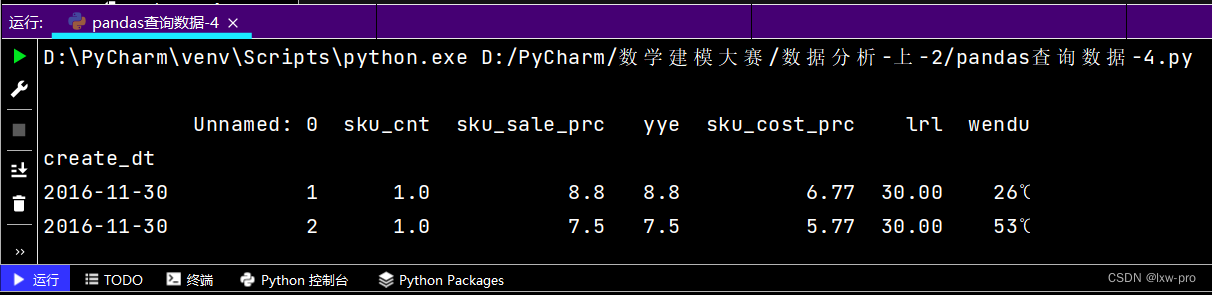# 查询数据类型
print(df.dtypes)

# 打印文件前几行


### 2-4-1 使用单个label值查询数据


print(df.loc['2016-12-02', 'yye'])   # 得到指定时间里相对应的的单个值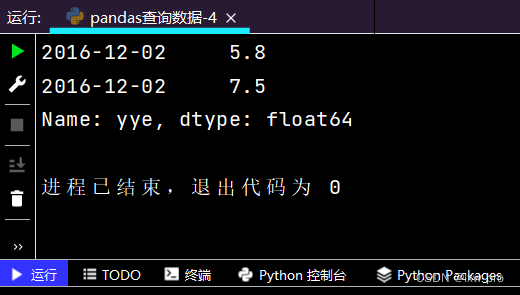# 得到指定时间内相对应的的一个Series
print(df.loc['2016-11-30', ['sku_cost_prc', 'sku_sale_prc']])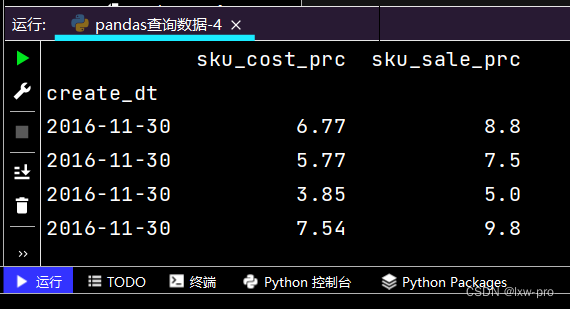### 2-4-2使用值列表批量查询

# 得到Series
print(df.loc[['2016-12-05', '2016-12-31'], 'sku_sale_prc'])


提示：图有点长，故只截取了部分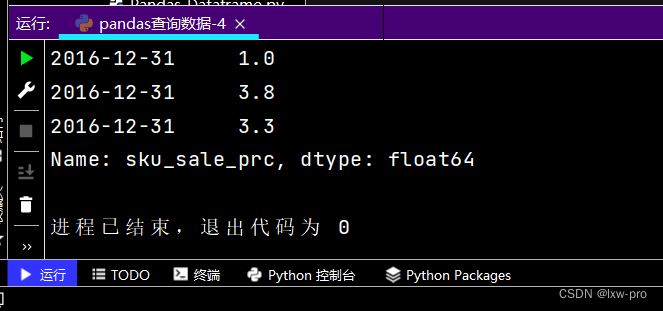# 得到DataFrame
print(df.loc[['2016-12-08', '2016-12-12'], ['sku_cnt', 'sku_sale_prc']])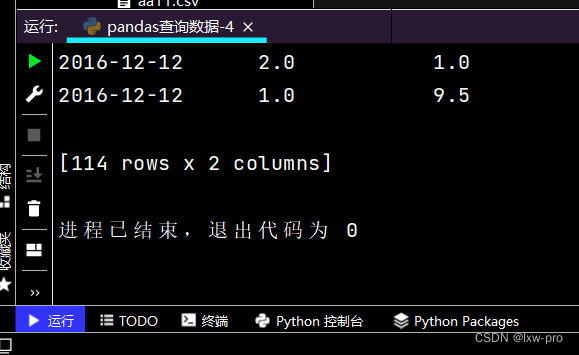### 2-4-3 使用数值区间进行范围查询

# 行index按区间：
print(df.loc['2016-12-02': '2016-12-08'], ['yye'])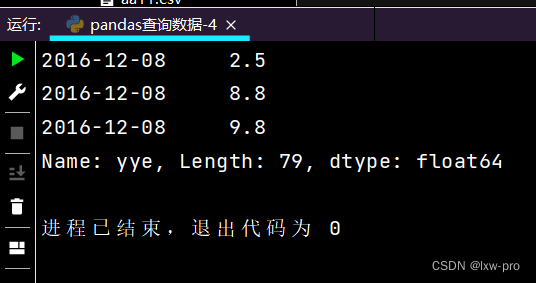# 列index按区间：
print(df.loc['2016-12-12', 'yye': 'lrl'])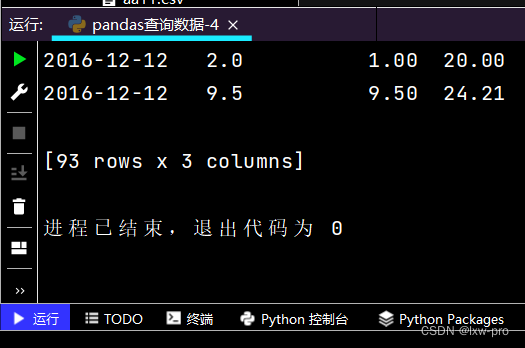# 行和列都按区间查询：
print(df.loc['2016-11-30': '2016-12-02', 'sku_cnt': 'lrl'])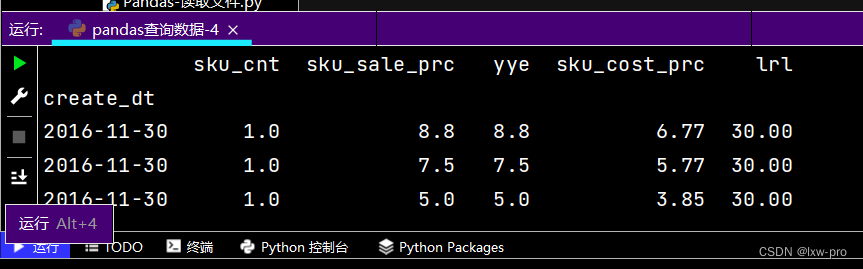### 2-4-4 使用条件表达式查询


# 简单条件查询，营业额低于3的列表
print(df.loc[df['yye'] < 3, :])

# 可观察营业额的boolean条件
print(df['yye'] < 3)

# 复杂条件查询：
print(df.loc[(df['yye'] < 5) & (df['yye'] > 2) & (df['sku_cnt'] > 1), :])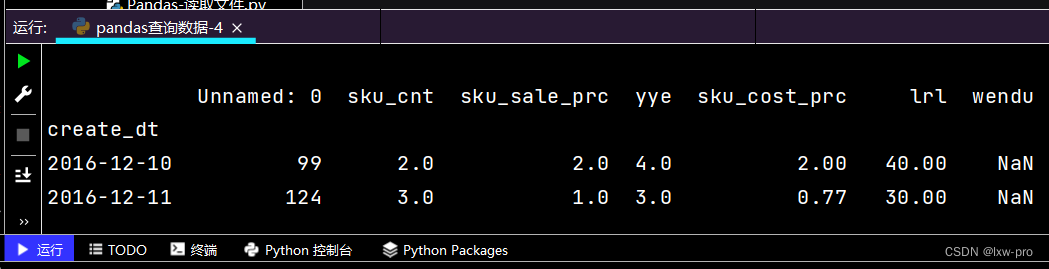# 再次观察这里的boolean条件
print((df['yye'] < 5) & (df['yye'] > 2) & (df['sku_cnt'] > 1))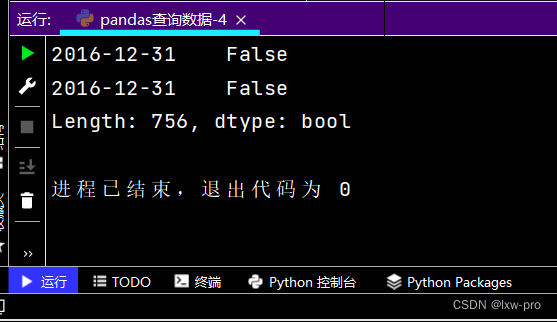### 2-4-5 调用函数查询

# 直接写lambda表达式
print(df.loc[lambda df: (df['yye'] < 4) & (df['yye'] > 2), :])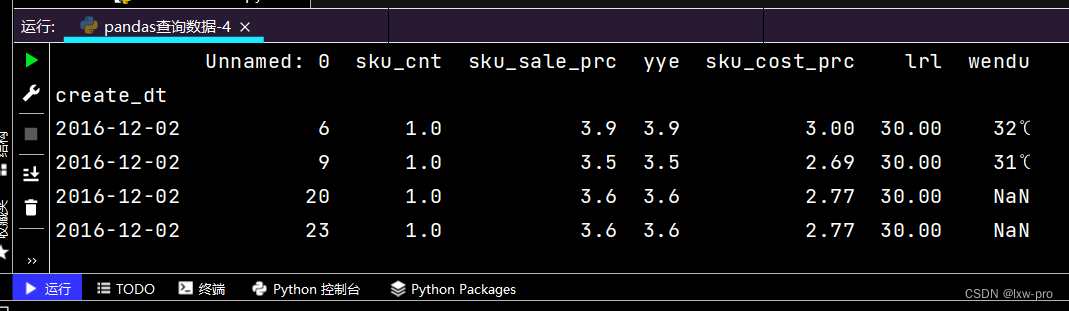# 函数式编程的本质：
# 函数本身可以像变量一样传递

def my_query(df):
return df.index.str.startswith('2016-12-08')

print(df.loc[my_query, :])


__

### 遇到的问题：

1、虽说三大模型十大算法【简介】讲的很是明确，可在网上要查询相关模型或者算法还是很杂乱的，不是很清楚自己适合那一版本。
2、学习pandas过程当中遇到查询数据时遇【替换掉利润率当中的后缀%】 出现差错，后面通过网上查询解决问题。

## 总结：

### 每日一言：

06-28554
08-111152
11-041万+
09-13493
11-15540
04-20

### “相关推荐”对你有帮助么？

•非常没帮助
•没帮助
•一般
•有帮助
•非常有帮助被折叠的  条评论 为什么被折叠?到【灌水乐园】发言lxw-pro

¥2 ¥4 ¥6 ¥10 ¥20余额支付 (余额：-- )扫码支付获取中扫码支付点击重新获取扫码支付1.余额是钱包充值的虚拟货币，按照1:1的比例进行支付金额的抵扣。
2.余额无法直接购买下载，可以购买VIP、C币套餐、付费专栏及课程。余额充值